#Factoring: Some Special Cases#### The binomial formulas.

Here are three algebraic formulas, the binomial formulas, which can be used for factoring: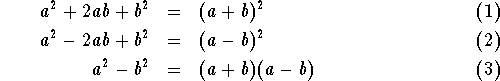You should check that these formulas work by multiplying out using the FOIL method.

Here is how to use these formulas for factoring purposes: Let's say we want to factor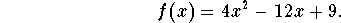We can write this polynomial as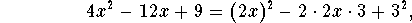and then notice that the terms match the second binomial formula for the values a=2x and b=3. Consequently,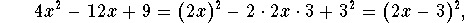and we have factored the polynomial completely. Note that x=3/2 is the only root, with multiplicity 2.

Here is another example: factor the polynomial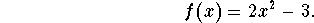We can write the polynomial as the difference of two squares and then use the third binomial formula: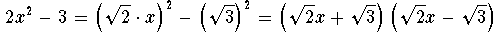#### The cube of a binomial.

There are similar formulas to factor some special cubic polynomials: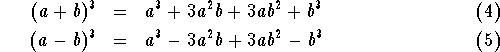As an example, let us factor the polynomial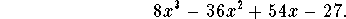We can rewrite this polynomial as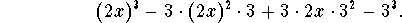Now it matches formula (5) with a=2x and b=3. Consequently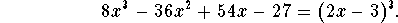The polynomial has a triple root at x=3/2.

#### Sums and differences of two cubes.

here are two more formulas to handle special cases of cubic polynomials: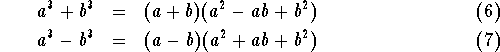Say, we like to factor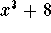. By formula (6), we can write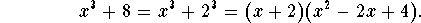In this case the factorization is complete, since the polynomial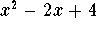is an irreducible quadratic polynomial.

What about the polynomial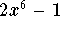? We first write this as the difference of two cubes, and then use formula (7):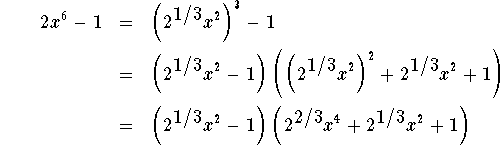Aside: Note that the factorization is still not complete. The Fundamental Theorem of Algebra tells us that it is possible to factor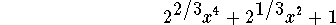further. Since you can see from the graph of this polynomial that it does not have real roots, the polynomialcan be factored into 2 irreducible quadratic polynomials. To find these two polynomials requires more familiarity with complex numbers; you can check that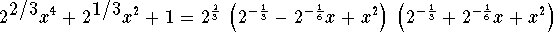#### Exercise 1.

Factor the polynomial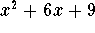completely.

#### Exercise 2.

Factor the polynomial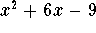completely.

#### Exercise 3.

Factor the polynomial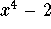completely.

#### Exercise 4.

Factor the polynomial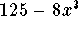completely.

#### Exercise 5.

Factor the polynomial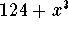completely.[Back] [Next]
[Algebra] [Trigonometry] [Complex Variables]
[Calculus] [Differential Equations] [Matrix Algebra]S.O.S MATHematics home page

Do you need more help? Please post your question on our S.O.S. Mathematics CyberBoard.Helmut Knaust